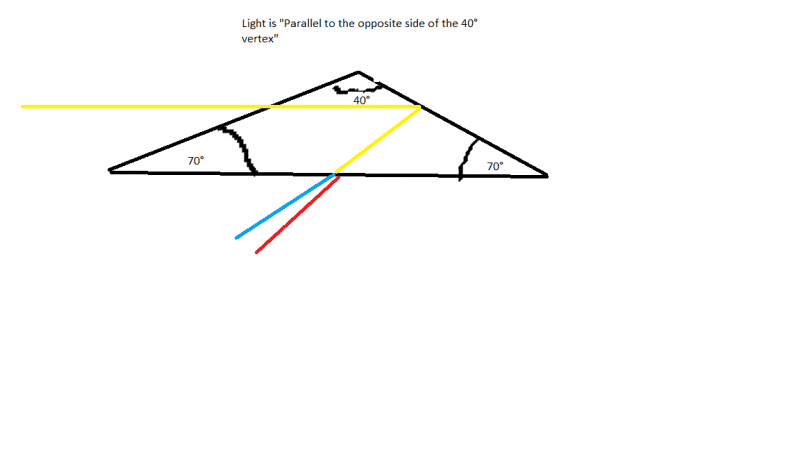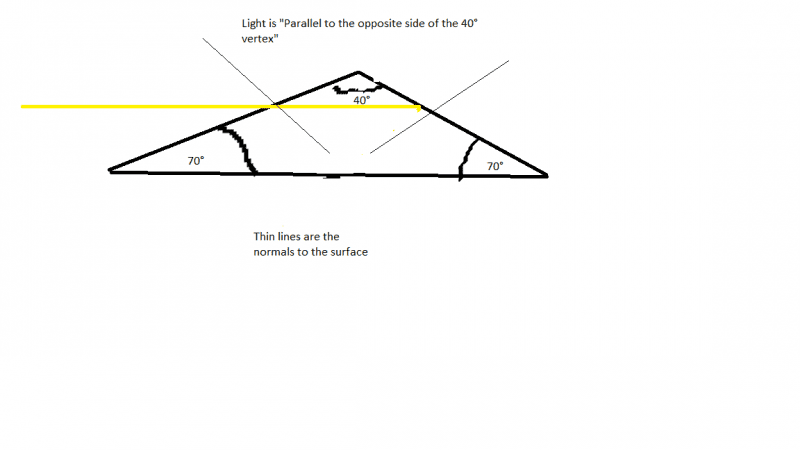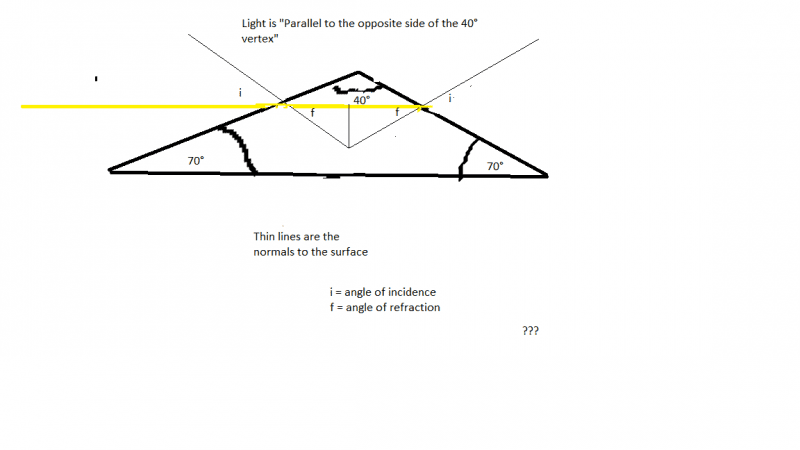# What is the angle between the two emerging beams of light?

## Homework Statement

Light consisting of a mixture of red and blue light enters a 40°, 70°, 70° prism along a line parallel to the side opposite the 40° vertex. The index of refraction of the prism material for blue light is 1.530, and for red light it is 1.525. What is the angle between the two emerging beams of light?

## Homework Equations

$n_{1}*sin(Θ_{1}) = n_{2}*sin(Θ_{2})$
$n_{red} = 1.525$
$n_{blue} = 1.530$
$∆Θ = Θ_{blue} - Θ_{red}$

## The Attempt at a Solution

This is dispersion, so the incident light creates two refracted light rays. So, by Snell's Law

$n*sin(Θ) = n_{red}*sin(Θ_{red})$
$n*sin(Θ) = n_{blue}*sin(Θ_{blue})$

Now here's the part I'm not entirely sure about but decided to go with anyways:
I assumed $Θ = 70^{°}$ was the angle of incidence and that the index of refraction of the prism is the same as glass $n = 1.52$

Plugging in I get
$Θ_{blue} = 69.5^{°}$
$Θ_{red} = 69.0^{°}$

Therefore,
$∆Θ = 0.5^{°}$

which is one of the answers! But...I'm not too confident about this result because I only assumed what Θ was and what the index of refraction of the prism was without exactly knowing why. So even if my answer's right I still don't completely understand what I did.

Could anyone help me out with this?

Doc Al
Mentor
But...I'm not too confident about this result because I only assumed what Θ was and what the index of refraction of the prism was without exactly knowing why.
Two things:
(1) The angles of incidence and refraction are measured from the normal to the surface.
(2) The light passes through two surfaces, thus two refractions must be considered.

Draw yourself a diagram!Here's the diagram I drew for the problem. I had it on paper so I re-drew in Paint.
Now I'm have doubts if that's even right, heh.

gneill
Mentor
Are you sure that there's total internal reflection at the second interface?

Are you sure that there's total internal reflection at the second interface?

On second thought, no actually. Truthfully I doodled that after looking at some the book's pictures of light bouncing off a droplet.

Is this better? It looks like it makes more sense this time around.gneill
Mentor
Better, yes. You might want to exaggerate the angle of refraction at the first surface a bit to reveal the geometry. You'll need the angle of incidence at the second interface.

Better, yes. You might want to exaggerate the angle of refraction at the first surface a bit to reveal the geometry. You'll need the angle of incidence at the second interface.gneill
Mentor
One problem. The beam of light will not stay horizontal. It will bend at the first interface (there is refraction after all). So your angle f will not be angle of incidence for the second interface.